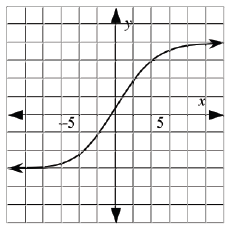### Home > CALC > Chapter 3 > Lesson 3.3.2 > Problem3-101

3-101.

Draw $f(x)$, given its slope statement.

The slope starts close to zero. When $x = −5$, the slope increases quickly. Then, at $x = 0$, the slope begins to decrease quickly until $x = 5$ when the slope is close to zero again.

Careful! This is a slope statement, not a description of $y$-values.